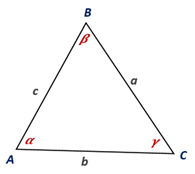Chapter 11.CT, Problem 16CTElementary Geometry For College St...

7th Edition
Alexander + 2 others
ISBN: 9781337614085

Solutions

Chapter
SectionElementary Geometry For College St...

7th Edition
Alexander + 2 others
ISBN: 9781337614085
Textbook Problem

On the basis of the drawing provided, complete the Law of Sines. sin ⁡ α a = _________To determine

To complete:

The law of sine using the drawing given.

Explanation

Given:

The Law of Sines.

Theorem:

In any acute triangle, the three ratios between the sines of the angles and the lengths of the opposite sides are equal and is given by sinαa=sin βb=sinγc or asinα=bsin β=csinγ, which is called the Law of Sines.

Calculation:

From the area formulas,

A=12bcsinα=12casinβ=12absinγ

Multiplying the areas by 2, we get

bcsinα=casinβ=absinγ

Dividing by abc, we get

bcsin⁡</

Still sussing out bartleby?

Check out a sample textbook solution.

See a sample solution

The Solution to Your Study Problems

Bartleby provides explanations to thousands of textbook problems written by our experts, many with advanced degrees!

Get Started

27. Find the number A that satisfies for the standard normal z-score.

Mathematical Applications for the Management, Life, and Social Sciences

Find f'(x) and f"(x). f(x)=xex

Single Variable Calculus: Early Transcendentals, Volume I

True or False: If , then converge absolutely.

Study Guide for Stewart's Single Variable Calculus: Early Transcendentals, 8th